# DAV Class 6 Maths Chapter 9 Worksheet 1 Solutions

The DAV Maths Book Class 6 Solutions and DAV Class 6 Maths Chapter 9 Worksheet 1 Solutions of Line Segments offer comprehensive answers to textbook questions.

## DAV Class 6 Maths Ch 9 WS 1 Solutions

Question 1.
Compare the following pairs of line segments using divider: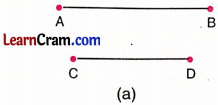(a) AB ____ CD
AB > CD(b) PQ ____ RS
PQ = RS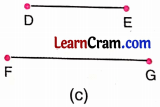(c) DE ____ FG
DE < FG

Question 2.
Measure the two line segments using the:
(a) Ruler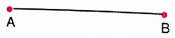(b) Divider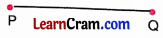Answer is not required. It is an activity to be done by the students themselves.Question 3.
Construct the line segments of the following lengths using compasses:
(a) 3.1 cm
(b) 6.2 cm
(c) 7.4 cm
(d) 9.3 cm
(a) 3.1 cm
Step 1: Draw a line l and take a point A on it.Step 2: Open the compass to a length of 3.1 cm and draw an arc B on the line.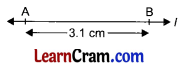Taking A as centre make a line segment AB.

(b) 6.2 cm
Step 1: Draw a line r and take a point P on it.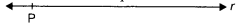Step 2: Open the compass upto 6.2 cm and with centre P, draw an arc Q making a line segment PQuestion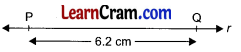Here, PQ is the required line segment of length 6.2 cm.

(c) 7.4 cm
Step 1: Draw a line m and take any point S on it.Step 2: Open the compass upto 7.4 cm and draw an arc T, taking S as centre making a line segment $$\overline{\mathrm{ST}}$$.Here, $$\overline{\mathrm{ST}}$$ is the required line segment of length 7.4 cm.

(d) 9.3 cm
Step 1: Draw a line q and take any point A on it.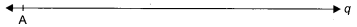Step 2: Open the compass upto 9.3 cm and draw an arc B, taking A as centre making a line segment $$\overline{\mathrm{AB}}$$.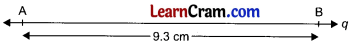Here, $$\overline{\mathrm{AB}}$$ is the required line segment of length 9.3 cm.

Question 4.
Construct the line segments equal in lengths to the segments given below:Step 1: Draw a line r and take any point P on it.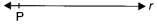Step 2: Open the compass and put in two pin point on the two ends of the given line segments A and B.
Step 3: Without disturbing the arms of the compass put its one pin on P and mark another arc at Q with its other arm pin making $$\overline{\mathrm{PQ}}$$ line segment.Here, PQ is the required line segment.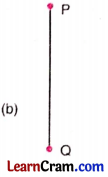Step 1: Draw a line s and take a point A on it.Step 2: Open the compass and put its two pin points on the two ends of the given line segment, P and Question
Step 3: Without disturbing the arms of the compass put its one pin on A and mark another arc at B with its other pin making AB line segment.Question 5.
Construct a line segment AB of length 7.5 cm. From this line segment, cut off a line segment AC of length 3.2 cm. Measure the length of the remaining line segment CB.Step 1: Draw line and take any point A on it.
Step 2: Open the compass upto 7.5 cm and put one pin point on A and mark an arc which cuts the line at B. So AB = 7.5 cm.
Step 3: Now again put the pin point of the compass on A and cut an arc equal to 3.2 cm and mark it as C.
Step 4:Measure the length of CB with the help of ruler or compass which is formed to be 4.3 cm.
Hence the length of the remaining segment = 4.3 cm.

Question 6.
If AB = 3.8 cm and CD = 2.5 cm, construct a line segment whose length is the sum of the lengths of these line segments and measure it.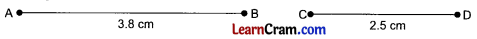Step 1: Draw a line and take any point P on it.
Step 2: Open the compass and measure the length AB.
Step 3: Put the pin-point of the compass at P and mark an arc at Q so that PQ = 3.8 cm.
Step 4: Put the pin-point of the compass at Q and mark an arc at R on the line at a distance of 2.5 cm.Step 5: $$\overline{\mathrm{PR}}=\overline{\mathrm{PQ}}+\overline{\mathrm{QR}}$$ = 3.8 cm + 2.5 cm = 6.3 cm Hence the required length is 6.3 cm.

Question 7.
If PQ = 5.4 cm and RS = 2.7 cm, construct a line segment whose length is the difference of the length of these line segments.Step 1 : Draw a line l and take a point A on it.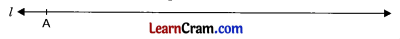Step 2: Using compass draw a line segment AB on line l equal to the length of given line PQ starting from point A.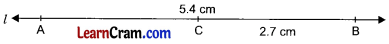Step 3: Now starting from point B (to the left) construct a line segment BC equal to the length of the given line segments RS with the help of compass.
Now AC is the required line segment.
$$\overline{\mathrm{AC}}=\overline{\mathrm{AB}}-\overline{\mathrm{CB}}$$ = 5.4 cm – 2.7 cm = 2.7 cm

Question 8.
If AB = 4.5 cm, CD = 2.3 cm, then construct the following line segments:
(a) 2 CD
2 CD
Step 1: Draw any line l and take any point R on it.
Step 2: Using compass starting from P construct a line segment PE equal to the length of CD i.e. 2.3 cm.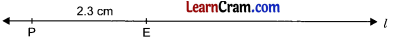Step 3: Starting from E draw a line segment EQ equal to the length of CD i.e.Hence PQ = PE + EQ
= 2.3 cm + 2.3 cm = 4.6 cm

(b) 3 AB
3 AB
Step 1: Draw any line l and take any point H on it.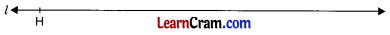Step 2: Using compass and starting from H, construct a line segment HT equal to the length of AB i.e. 4.5 cm.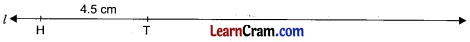Step 3: Now starting from T (to the right) draw a line segment TR equal to the length of AB i.e. 4.5 cmStep 4: Again starting from R(to the right) draw a line segment RS equal to the length of AB i.e. 4.5 cm.
Hence the required line segement is HS
where HS = HT + TR + RS
= 4.5 cm + 4.5 cm + 4.5 cm = 13.5 cm(c) AB – CD
AB – CD
Step 1: Draw any line l and take a point P on it.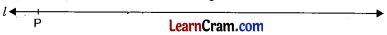Step 2: Using compass draw a line segment PQ on line l equal to the length of AB i.e. 4.5.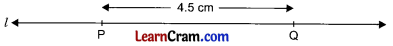Step 3: Now with the help of compass starting from Q (to the left), construct a line segment QS on l equal to the length CD i.e. 2.3 cm.Here PS is the line segment equal to the difference of AB – CD = 4.5 cm – 2.3 cm = 2.2 cm.

(d) AB + 2CD
AB + 2 CD
Step 1 : Draw any line l and take a point P on it.Step 2: Using compass, starting from P, draw a line segment PQ on l equal to the length of AB i.e. 4.5 cm.Step 3: Again using compass, starting from Q (to the right) construct a line segment QS on l equal to double the length of CD i.e. 2 x 2.3 = 4.6 cm.
Here PS is the required line segment
where PS = AB + 2 CD
= 4.5 + 2 × 2.3 = 4.5 + 4.6 = 9.1 cm

### DAV Class 6 Maths Chapter 9 Value Based Questions

Question 1.
A group of four children were given a project where they were asked to bring some eco-friendly fibres. Neha forgot to bring the fibre and was upset. Two of her friends Sneha and Swati shared their fibres with Neha. Sneha gave 16.5 cm of fibre and Swati gave 8.5 cm of fibre to her.
(a) How much more fibre did Sneha give to Neha than Swati? Construct the line segment representing this length.
(b) What value is exhibited by the children?
(a) Sneha gave 16.5 cm of fibre and Swati gave 8.5 cm of fibreDifference between lengths of fibres of Sneha and Swati is
= 16.5 cm – 8.5 cm = 8 cm
So, Sneha gave 8.0 cm more fibre to Neha than Swati.

(b) The value of cooperation is exhibited by the children.

### DAV Class 6 Maths Chapter 9 Worksheet 1 Notes

Line segment is a portion of a line having two end points. It has definite length. It is represented by $$\overline{\mathrm{AB}}$$.Example: AB is a segment. A and B are its two ends.

Comparison of line segments:
The two line segments can be compared by measuring their lengths with the help of divider.Here, we can observe that $$\overline{\mathrm{CD}}$$ is longer than $$\overline{\mathrm{AB}}$$ or $$\overline{\mathrm{AB}}$$ is shorter than $$\overline{\mathrm{CD}}$$.

Length of the line segment can be measured with the help of ruler or scale.